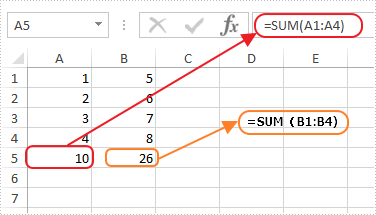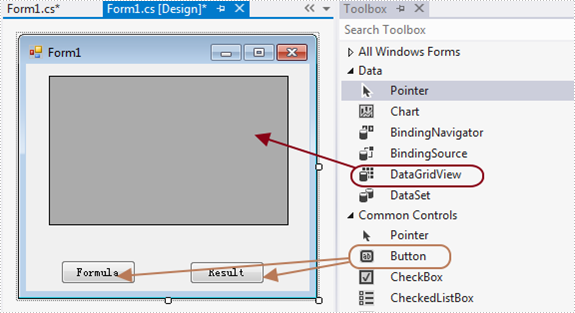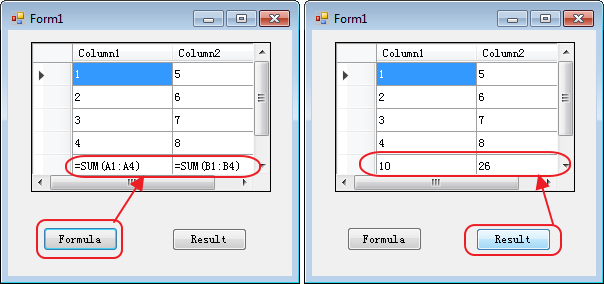# Show formula and its result separately when converting excel to datatable in C#

This article shows how to display formula and its result separately when converting excel to database via Spire.XLS. This demo uses an Excel file with formula in it and show the conversion result in a Windows Forms Application project.

Screenshot of the test excel file:Here are the detailed steps:

Steps 1: Create a Windows Forms Application in Visual Studio.

Steps 2: Drag a DataGridView and two Buttons from Toolbox to the Form and change names of the buttons as Formula and Result to distinguish.Steps 3: Double click Button formula and add the following code.

3.1 Load test file and get the first sheet.

```Workbook workbook = new Workbook();
Worksheet sheet = workbook.Worksheets;
```

3.2 Invoke method ExportDataTable of the sheet and output data range. Parameters of ExportDataTable are range to export, indicates if export column name and indicates whether compute formula value, then it will return exported datatable.

Description of ExportDataTable:

```public DataTable ExportDataTable(CellRange range, bool exportColumnNames, bool computedFormulaValue);
```

Code:

```DataTable dt = sheet.ExportDataTable(sheet.AllocatedRange, false, false);
```

3.3 Show in DataGridView

`this.dataGridView1.DataSource = dt; `

Steps 4: Do ditto to Button Result. Only alter parameter computedFormulaValue as true.

```Workbook workbook = new Workbook();
Worksheet sheet = workbook.Worksheets;
DataTable dt = sheet.ExportDataTable(sheet.AllocatedRange, false, true);
this.dataGridView1.DataSource = dt; ```

Steps 5: Start the project and check the result.Button code here:

```//Formula
private void button1_Click(object sender, EventArgs e)
{
Workbook workbook = new Workbook();
Worksheet sheet = workbook.Worksheets;
DataTable dt = sheet.ExportDataTable(sheet.AllocatedRange, false, false);
this.dataGridView1.DataSource = dt;

}
//Result
private void button2_Click(object sender, EventArgs e)
{
Workbook workbook = new Workbook();